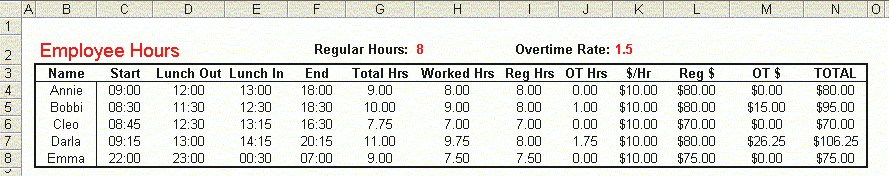Working With Overtime Hours In Excel

 Excel is often used to manage employee time records -- number of hours worked, meal breaks, regular and overtime pay, and so on.  This page examines a number of related formulas for a simple daily timekeeping record.    Suppose we have 5 employees, and we want to keep track of the time each comes into work, breaks for lunch, returns from lunch, and leaves for the day.  Also, we need to keep track of the regular and overtime hours for each employee, and pay the the overtime hours at a different rate.  The sample below shows what the worksheet might look like:Now, lets look at the values and formulas in each column.  Column B is simply the Name of each employee.  Columns C, D, E, and F are the times that each employee started work, left for lunch, returned from lunch, and left work for the day.   Pay attention to the hours worked by Emma, in row 8.  Her shift extended over midnight (she started at 10PM and left at 7AM), and her lunch break also extended over midnight.  Our formulas will take this into account, and calculate the proper times.  Cell H2 contains the number of regular, non-overtime, hours per shift.  Here, the 8 indicates that a normal shift is 8 hours.  Cell K2 contains the rate at which overtime hours are paid.  The 1.5 indicates that overtime is paid at time-and-a-half.    The total hours are in column G.  This is the difference between the start time in column B and the end time in column F.  This value does not pay any attention to the time taken for lunch -- we'll do that later.  The formula in G4 is:  =(F4-C4+(F4You can download a workbook with these formulas.  Additional Resources: John Walkenbach has an example timesheet here. David McRitchie has an example timesheet here.CalculateHours.com has a library of many different Excel-based timesheet that you can use as they are or you can customize to suit your particular needs.
 Other Date And Time Related Procedures are described on the following pages. Adding Months And Years The DATEDIF Function Date Intervals Dates And Times Date And Time Entry Date And Time Arithmetic Distributing Dates Over Intervals Holidays Julian Dates Latitude And Longitude  Overtime Hours And Timesheets VBA Procedures For Dates And Times Week Numbers Worksheet Functions For Dates And Times See the Dates And Times Topic Index For Information